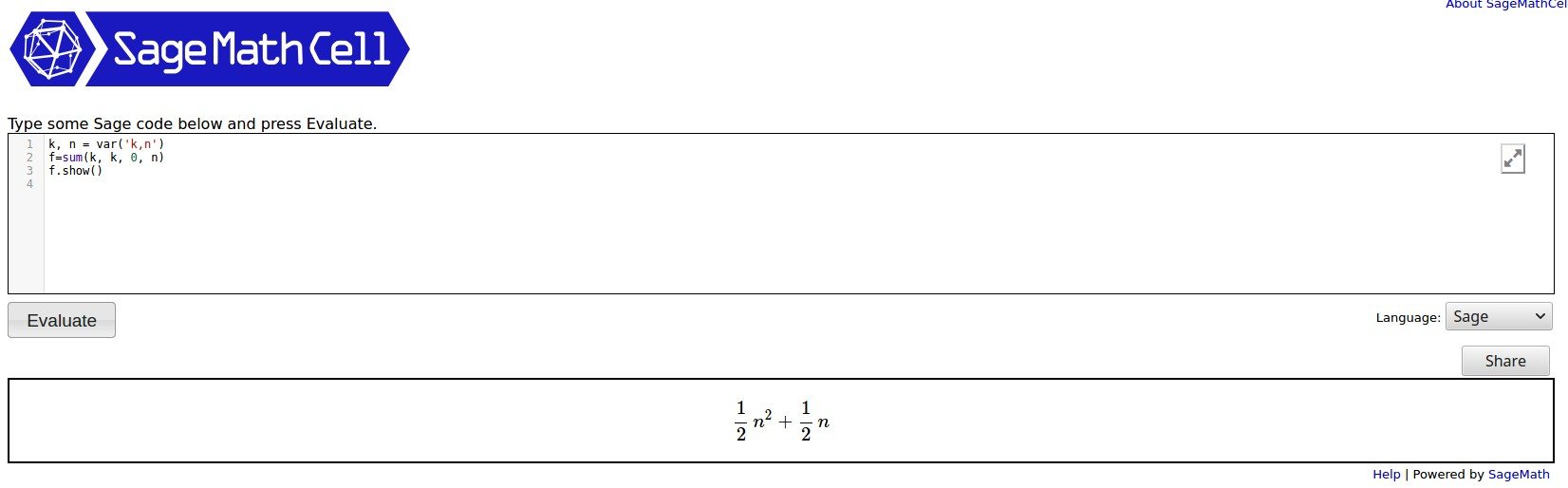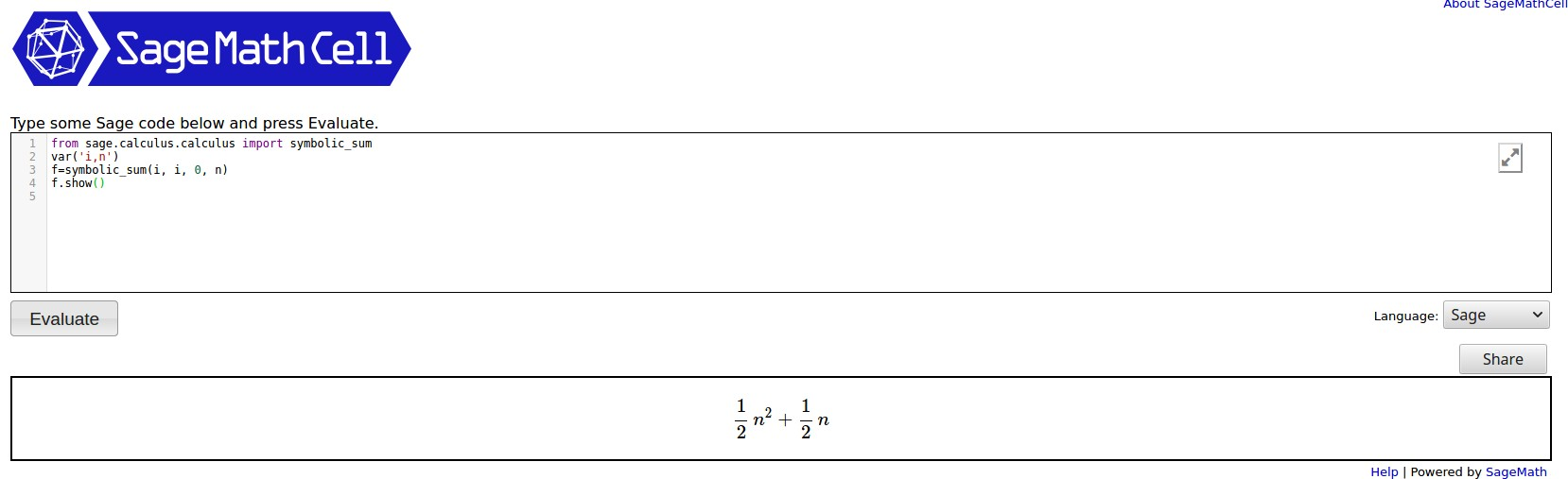# Simple question on displaying summation

Hi,

I'm defining a few summation functions for displaying and evaluating later, but I don't understand how to do it.

So, I'm looking at the basic Sage Quick Reference and I see under Arithmetic: sum(f(i) for i in (k..n))

But when I try to make it symbolic to show, like something simple like this:

var('i,n')
f(n)=sum(i for i in (0..n))
show(f)


But then I get

TypeError: unable to simplify to float approximation


Which makes me think its trying to evaluate the sum rather than make it a symbolic expression. Then I ran across "symbolic_sum" somewhere, but I can't find any documentation about it now...

Any suggestions?

Erik

edit retag close merge delete

Sort by » oldest newest most voted

If you want a symbolic sum you have 2 choices that I know of. First, using sum:

k, n = var('k,n')
f=sum(k, k, 0, n)
f.show()


The output, running in a SageCellServer is shown below:You can find the documentation here.

If you use symbolic_sum, documented here, then you could try:

from sage.calculus.calculus import symbolic_sum
var('i,n')
f=symbolic_sum(i, i, 0, n)
f.show()


The output is:more

Ok thanks for the answer. I guess if I want to display it in pretty summation notation I will need to use LaTeX. Glad I wasn't missing anything.

I don't think that can be done using just Sage. When I combine LaTeX and Sage, I do it as a document using the sagetex package in LaTeX. The CTAN documentation is here.